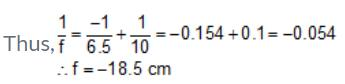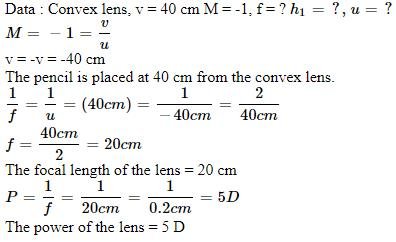Courses

# Test: Lense Formula

## 10 Questions MCQ Test Science Class 10 | Test: Lense Formula

Description
This mock test of Test: Lense Formula for Class 10 helps you for every Class 10 entrance exam. This contains 10 Multiple Choice Questions for Class 10 Test: Lense Formula (mcq) to study with solutions a complete question bank. The solved questions answers in this Test: Lense Formula quiz give you a good mix of easy questions and tough questions. Class 10 students definitely take this Test: Lense Formula exercise for a better result in the exam. You can find other Test: Lense Formula extra questions, long questions & short questions for Class 10 on EduRev as well by searching above.
QUESTION: 1

### An object is 9 cm from a magnifying lens and its image is formed 36 cm from the lens. Magnification of the lens is

Solution:

Magnification = the height of the image ÷ by the height of the object
M=36/9 =4

QUESTION: 2

### An object 4 cm tall is placed in front of a convex lens. It produces an image 3 cm tall. What is the magnification of the lens ?

Solution:

We know, height of the object is 4 cm h1, height of the image is 3 cm, h2.
So we have, m = h2/h1
=> m = ¾ m
=> m = 0.75 .
Therefore, magnification of the lens is 0.75

QUESTION: 3

### A convergent beam of light passes through a diverging lens of focal length 0.2 m and comes to focus 0.3 m behind the lens. Find the position of the point at which the beam would converge in the absence of lens.​

Solution:

72.f = - 0.2 m, v = + 0.3 mThe lens formula 1v - 1u = 1f
1u = 1v - 1f = 10.3 + 10.2
= 0.50/006
u = 0.12 m

QUESTION: 4

An object is placed 10 cm from a diverging lens which forms an image 6.5 cm from the lens. What is the focal length of the lens ?

Solution:

Since, it is a diverging lens, that is, a concave lens, we have
u = -10 cm, v = -6.5 cmQUESTION: 5

A convex lens of focal length 12 cm produces a magnification of -1. The object should be placed at;​

Solution:

1/v - 1/u
1/f   =-1/u - 1/u
1/f   = - 2/u
u=-2f
u=-24 cm

QUESTION: 6

An object is situated at a distance of f/2 from a convex lens of focal length f. Distance of image will be

Solution:

If the object is placed at distance of f/2 in front of convex lens of focal length 'f'.
If the object is at f/2 distance then it means it is between the focus and optical centre of lens. And we know, that when an object is placed between the focus and optical centre of convex lens then the image is formed behind the object and the nature of image is virtual, errect and magnified.
By lens formula ;
1/f = 1/v - 1/u
1/f  = 1/v - 1/ (-f/2)
Solving this we get,
1/ v = -f/2 /  f2/2
v = f2 /2 / -f/2 = -f

QUESTION: 7

A convex lens forms a real, inverted and same sized image as the object placed at a distance of 40 cm from it. The power of the lens is _____D.

Solution:QUESTION: 8

A concave lens of 20 cm focal length forms an image 15 cm from the lens. What is the object distance ?

Solution:

Focal length= -20(as it is concave lens)
v= -15 (as concave lens always forms virtual and erect image on left of lens)
Putting these values in lens formula,
1/ -20 - 1/u = 1/ -15
-1/ u= 1/-15 + 1/20
-1/u = -4+3/60
-1/u = -1/60
-u = -60
[u =60]

QUESTION: 9

Where should an object be placed in front of convex lens so as to obtain the image formed on slide projector ?​

Solution:

An object should be placed between F1 and 2 F1 so as to obtain the image formation used in slide projector. This is because, the image has to be enlarged and has to form at a little larger distance from the projector.

QUESTION: 10

The distance at which an object should be placed from a thin convex lens of focal length 10 cm to obtain a virtual image of double of its size is​

Solution:

Given : f = 10 cm , m = 2
v / u = 2
v = 2u
v = 2u -------(1)
according to lens formula ,
1/v - 1/u = 1/f
1/v - 1/u = 1/10 --------(2)
substitute (1) in (2)
1/2u - 1/u = 1/10
1 - 2 / 2u = 1/10
-1 / 2u = 1/10
-10 = 2u
u = -10/2
u = -5 cm
Therfore object should be placed 5 cm away from the lens.